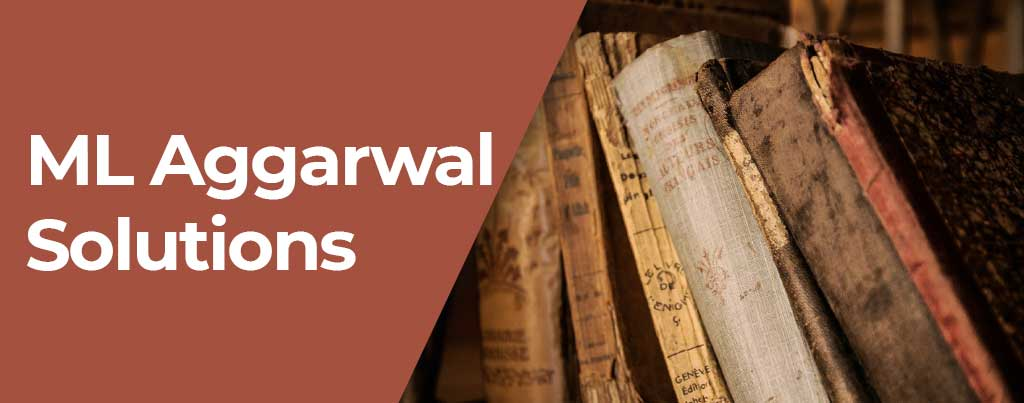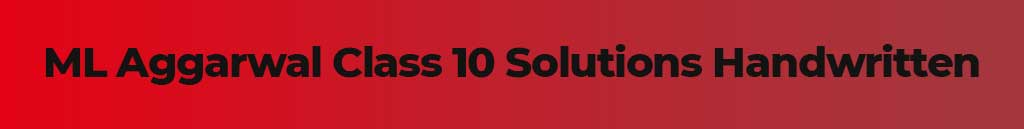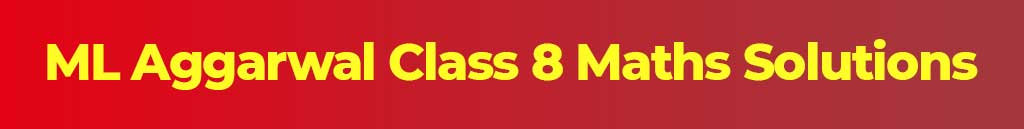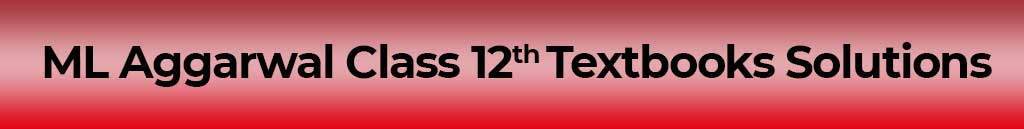# ML Aggarwal SolutionsML Aggarwal Solutions during these years show its growing demand among students and teachers. The ML Aggarwal Maths has been revised thoroughly again strictly conforming to the latest syllabus pattern issued by the Council for ICSE board. The latest feature “Multiple Choice Questions” has been added in every chapter of ML Aggarwal Solutions. At the end of every chapter, there is a Test which gives the students a complete knowledge of the topics discussed.

ML Aggarwal Solutions has been designed keeping in mind the age group of the students who will surely find it very user-friendly. ML Aggarwal Solutions has been divided into 2 parts. So that students can learn at their own pace. These Solutions has been given in an easy language and it induces many examples from real-life conditions. Emphasis has been laid on basic terms, concepts, principles and their applications.

Carefully selected examples are provided with step-by-step ML Aggarwal Solutions. A number of solved examples are covered in each section to enable the students to take all the problems given in the lessons.## ML Aggarwal Class 10 Solutions HandwrittenML Aggarwal Class 10 Solutions in Handwritten are available here. Students willing to solve the exercise questions given in the mathematics book of 10th standard can refer to this ML Aggarwal Class 10 ICSE Solutions. It is an ideal resource to prepare for the board exams and score higher marks.

These are developed by the mathematics experts, they have done a rigorous study on the topics given in the std 10 maths book. The solutions that we are providing here are curated in a way that will help the learners to cross check their given answers easily.

The questions and answers are presented in a way that aids in comprehending them effortlessly. ML Aggarwal Class 10 Maths Solutions PDF can be downloaded from the below given links. It contains chapter wise solutions for a total of 22 chapters.

ICSE Class 10 ML Aggarwal solutions Chapter Wise## ML Aggarwal Class 9 Maths SolutionsML Aggarwal Class 9 Maths Solutions can be used by the students in order to solve the exercise problems and cross check their written answers. The topics that are elaborated in std 9th are essential because in future classes such as in 10th, these topics will work like a base. Without having a good understanding of class 9th mathematics, clearing the further standards will be quite difficult. Thus, referring to the ML Aggarwal Class 9 Solutions can be very helpful. This improves the student’s confidence to appear in the annual exam of class 9th without having any fear.

The answers that are created in it are in stepwise format. Anyone who is struggling to find out the right steps to reach out to the correct answer can use these solutions without any tension.

The links that will aid the 9th standard learners to download ML Aggarwal Class 9 ICSE Solutions are given below in chapter wise manner.

ICSE Class 9 ML Aggarwal solutions Chapter Wise

Chapter 1 Rational and Irrational Numbers

Chapter 2 Compound Interest

Chapter 3  Expansions

Chapter 4  Factorization

Chapter 5  Simultaneous Linear Equations

Chapter 6  Problems on Simultaneous Linear Equations

Chapter 8  Indices

Chapter 9  Logarithms

Chapter 10 Triangles

Chapter 11 Mid Point Theorem

Chapter 12  Pythagoras Theorem

Chapter 13  Rectilinear Figures

Chapter 14  Theorems on Area

Chapter 15  Circle

Chapter 16  Mensuration

Chapter 17  Trigonometric Ratios

Chapter 18  Trigonometric Ratios and Standard Angles

Chapter 19  Coordinate Geometry

Chapter 20  Statistics## ML Aggarwal Class 8 Maths SolutionsML Aggarwal Class 8 Maths Solutions works as the best reference materials for the final exam preparation. It is one of the best methods to explore and solve a variety of questions. The ML Aggarwal Class 8 ICSE Solutions are given here, it includes all the chapters and exercise questions thoroughly. The solutions of all those questions have been explained in full detail with the help of stepwise explanation.

By using this, students can practice the plethora of exercise questions. It will improve their understanding and boost their confidence to overcome their fear of mathematics. These solutions are provided in very simple and clear languages.

ICSE Class 8 ML Aggarwal solutions Chapter Wise

Chapter 1 Rational Numbers

Chapter 2 Exponents and Powers

Chapter 3 Squares and Square Roots

Chapter 4 Cubes and Cube Roots

Chapter 5 Playing with Numbers

Chapter 6 Operation on sets Venn Diagrams

Chapter 7 Percentage

Chapter 8 Simple and Compound Interest

Chapter 9 Direct and Inverse Variation

Chapter 10 Algebraic Expressions and Identities

Chapter 11 Factorisation

Chapter 12 Linear Equations and Inequalities In one Variable

Chapter 15 Circle

Chapter 16 Symmetry Reflection and Rotation

Chapter 17 Visualising Solid Shapes

Chapter 18 Mensuration

Chapter 19 Data Handling## ML Aggarwal Class 7 Maths SolutionsML Aggarwal Class 7 Maths Solutions which can be accessed here on this website is free available in PDF format. It can assist the students to learn different types of mathematics tips, tricks and shortcuts to solve any maths questions that is prescribed to the std 7th students. The solutions are referred to the students to get the deep insights to the answers and its methods.

It emphasizes the learnings of students by giving them well prepared solutions prepared by subject matter experts. The ML Aggarwal Solutions helps students to keep in sync with the learnings and try new methods to solve the mathematics questions. The solutions are in a manner that enables the candidates to answer the questions in the exam in the most effective way.

ICSE Class 7 ML Aggarwal solutions Chapter Wise

Chapter 1 Integers

Chapter 2 Fractions and Decimals

Chapter 3 Rational Numbers

Chapter 4 Exponents and Powers

Chapter 5 Sets

Chapter 6 Ratio and Proportion

Chapter 7 Percentage and Its applications

Chapter 8 Algebraic Expressions

Chapter 9 Linear Equations and Inequalities

Chapter 10 Lines and Angles

Chapter 11 Triangles and its Properties

Chapter 12 Congruence of Triangles

Chapter 13 Practical Geometry

Chapter 14 Symmetry

Chapter 15 Visualising Solid Shapes

Chapter 16 Perimeter and Area

Chapter 17 Data Handling## ML Aggarwal Class 6 Maths SolutionsML Aggarwal Class 6 Maths Solutions helps students to make their basic and fundamental knowledge stronger. Class 6th is the base standard of all the upcoming classes such as 7, 8, 9, 10, etc. Therefore, it is essential to grasp the concepts of mathematics rigorously. The ML Aggarwal solutions that are given here fulfill that requirement and it helps in daily practice of Class 6 ML Aggarwal questions.

The daily practice of it, helps students in improving their time management, analytical skills and thinking ability. From the exam perspective these things are essential and aid in getting higher scores in the annual exams.

To strengthen the class 6th mathematics conceptual knowledge students can refer to the below given links to download the ML Aggarwal solutions for class 6th.

ICSE Class 6 ML Aggarwal solutions Chapter Wise

Chapter 1 Knowing Our Numbers

Chapter 2 Whole Numbers

Chapter 3 Integers

Chapter 4 Playing with Numbers

Chapter 5 Sets

Chapter 6 Fractions

Chapter 7 Decimals

Chapter 8 Ratio and Proportion

Chapter 9 Algebra

Chapter 10 Basic Geometrical Concept

Chapter 12 Symmetry

## Why Should Students Follow ML Aggarwal Solutions?Students should follow ML Aggarwal Solutions to improve their understanding of Important mathematics concepts. Along with that it is based on the basis of ICSE Board Syllabus. So referring to these will boost the exam preparation process. It also gives them an opportunity to explore and expand their basic knowledge of mathematics.

To grasp the concepts faster and quicker the use of solutions books can be considered best. It is because the solutions provide the clear and to the point answer to all the questions available in each chapter. It also aids in coping up with the difficult questions effortlessly.

While preparing for the board exam or normal classroom exams the solutions will always support learners to learn and improve. That is why students should Follow ML Aggarwal Solutions as it is ideal and best for ICSE Board students.## ML Aggarwal Class 12th Textbooks SolutionsML Aggarwal Class 12th Textbooks Solutions helps the board candidates to deal with various difficult and advanced questions given in the books. The solutions are essential resources that aid in the learning and exam preparation simultaneously.

However, to prepare the ML Aggarwal Class 12th Mathematics Solutions they will need ML Aggarwal mathematics textbooks. We are given the direct download links in the below given section. It is present in chapter wise format.

ML Aggarwal 12th Class Maths Books - Sections A

Chapter 1 Determinants

Chapter 2 Matrices

Chapter 3 Boolean Algebra

Chapter 4 Conics

Chapter 5 Inverse Trigonometric Functions

Chapter 6 Differentiation

Chapter 7 Indeterminate Forms

Chapter 8 Mean Value Theorems Maxima Minima

Chapter 9 Integration

Chapter 10 Definite Integrals

Chapter 11 Applications Of Definite Integrals

Chapter 12 Correlation And Regression

Chapter 13 Probability

Chapter 14 Complex Numbers

Chapter 15 Differential Equations

ML Aggarwal 12th Class Maths Books - Sections B

Chapter 1 Vectors

Chapter 2 Straight Lines In Space

Chapter 3 Planes

Chapter 4 Probability

ML Aggarwal 12th Class Maths Books - Sections C

Chapter 1 Discount

Chapter 2 Annuities

Chapter 3 Linear Programming

Chapter 4 Application of Derivatives in Commerce And Economics

Chapter 5 Index Numbers And Moving Averages## ML Aggarwal Class 11 Maths Textbook SolutionsML Aggarwal Class 11 Maths Solutions can be quite helpful to solve and practice the number of questions given in them. All the concepts need to be thoroughly explained with the support of tips, tricks and the given formulas. For making a solution that can benefit the students in numerous ways they need to have a Class 11th ML Aggarwal textbook.

Here on this webpage, we have provided the complete set of books that will help the candidates to practice more and more mathematics questions. It will also help them in making the short notes and the solutions books for the exam preparation purposes. The below given links are the direct link to access the ML Aggarwal Class 11 Maths Textbook chapter wise for free of cost.

ML Aggarwal Class 11 Maths Textbook Chapter Wise

Chapter 1 Sets

Chapter 2 Relations And Functions

Chapter 3 Trigonometric Functions

Chapter 4 Principle Of Mathematical Induction

Chapter 5 Complex Numbers And Quadratic Equations

Chapter 6 Linear Inequalities

Chapter 7 Permutations And Combinations

Chapter 8 Binomial Theorem

Chapter 9 Sequences And Series

Chapter 10 Straight Lines

Chapter 11 Conic Sections

Chapter 12 Introduction To Three Dimensional Geometry

Chapter 13 Limits And Derivatives

Chapter 14 Mathematical Reasoning

Chapter 15 Statistics

Chapter 16 Probability

### Key Features of ML Aggarwal Solutions:• Designed by subject experts
• Easy & comprehensive language
• Act as step-by-step solutions
• Cover all the subjects for Class 9th & 10th
• Easy online accessibility
• Available free of cost
• Created according to the latest ICSE syllabus pattern

## You Can Download Here ML Aggarwal Maths Books and Solutions for Class 6th to 12th### FAQ on ML Aggarwal Solutions for ICSEFAQs×